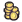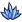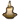Several scores are calculated throughout the games. You can see them at the end of a match by opening the statistics tab.

# Military score

Calculated as the sum of the value of your units calculated as (to which a baseline value of 1 is added):

[production cost of the unit]+[production cost of the unit]*2
For Goblins and Dwarves, the production cost does not take into account the -10% and +10% modifier (so a Goblin Marauder for instance has a Production cost of 45 for score matters, and not 40 as is actually the case).

and Heroes have a value of 50, whatever their level.

# Knowledge score

Calculated the sum of theof all the skills you have researched or aquired.

# Domain score

Has a minimum value of 50, even if you have no domain left.
Depends on the number of cities with different radius, as well as population and building values.

# General score

Calculated as the sum of the other scores: Military + Knowledge + Domain + Happiness + Seal.